Courses

# Major Inorganic Chemistry

## 60 Questions MCQ Test MOCK Test Series for IIT JAM Chemistry | Major Inorganic Chemistry

Description
This mock test of Major Inorganic Chemistry for Chemistry helps you for every Chemistry entrance exam. This contains 60 Multiple Choice Questions for Chemistry Major Inorganic Chemistry (mcq) to study with solutions a complete question bank. The solved questions answers in this Major Inorganic Chemistry quiz give you a good mix of easy questions and tough questions. Chemistry students definitely take this Major Inorganic Chemistry exercise for a better result in the exam. You can find other Major Inorganic Chemistry extra questions, long questions & short questions for Chemistry on EduRev as well by searching above.
QUESTION: 1

Solution:
QUESTION: 2

Solution:
QUESTION: 3

### The correct order of boiling point is:

Solution:
QUESTION: 4

MnCr2O4 is:

Solution:
QUESTION: 5

Silica gel contains [CoCl4]2– as an indicator when activated, silica gel becomes dark blue while upon absorption of moisture, its color changes to pale pink. This is because:

Solution:
QUESTION: 6

The HOMO in CO is:

Solution:
QUESTION: 7

What is the geometry of [AuCl4] ion?

Solution:
QUESTION: 8

The reagent ‘oxine’ commonly used in analytical chemistry is:

Solution:
QUESTION: 9

Thermal stability of hydrides of first group elements follows the order

Solution:

As we move from top to bottom in a group the size of the alkali metals increases, thereby the bond dissociation energy decreases hence it requires less energy to decompose so thermal stability also decreases..
So the order of thermal stability of IA group elements is

LiH > NaH > KH > RbH.

QUESTION: 10

On dissolving moderate amount of sodium metal in liquid NH3 at low temperature, which one of the following does not occur?

Solution:
QUESTION: 11

Mg2C3 reacts with water forming propyne, C4-3 has:

Solution:
QUESTION: 12

At pH 7, the zinc(II) ion in carbonic anhydrase reacts with CO2 to give:

Solution:
QUESTION: 13

NH4Cl (s) is heated in test tube. Vapours are brought in contact with red litmus paper, which  changes to blue and then to red. It is because of :

Solution:
QUESTION: 14

Each of the compounds Pt(NH3)6Cl4, Cr(NH3)6Cl3, Co(NH3)4Cl3 and K2PtCl6 were dissolved in water to make its 0.001 M solution. The correct order of their increasing conductivity in solution is:

Solution:
QUESTION: 15

Hot concentrated HNO3 converts graphite into :

Solution:
QUESTION: 16

BH3.CO in more stable than BF3.CO because:

Solution:
QUESTION: 17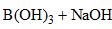⇌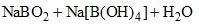How can this reaction be made to proceed in forward direction?

Solution:
QUESTION: 18

In cyclophosphazenes, (NPX2)3 (X = F, Cl, Br and Me), the strength of p—N p-bond various with X in the order:

Solution:
QUESTION: 19

Which of the following statemets is true?

Solution:
QUESTION: 20

Toxicity of cadmium and mercury in the body in being reversed by proteins, mainly using the amino acid residue:

Solution:
QUESTION: 21

For the following reaction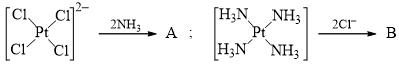The major product A and B are respectively:

Solution:
QUESTION: 22

The spin-only (μS) and spin plus orbital (μS+L) magnetic moment of [CrCl6]3– are:

Solution:
QUESTION: 23

The red colour of ruby is due to:

Solution:
QUESTION: 24

The carbide which gives ethyne on hydrolysis:

Solution:
QUESTION: 25

Choose the correct option for carbonyl fluoride with respect to bond angle and bond length:

Solution:
QUESTION: 26

Which of the following is false?

Solution:
QUESTION: 27

Strength of hydrogen bond is intermediate between:

Solution:
QUESTION: 28

Correct order of strength of bonding among: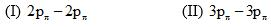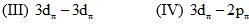Solution:
QUESTION: 29

Polarization is the distortion of the shape of an anion by an adjacently placed cation. Which of the following statement is correct:

Solution:
QUESTION: 30

Correct order of P—P bond length in the following compound is:

Solution:
*Multiple options can be correct
QUESTION: 31

In which of the following reaction(s) hybridization of asterisk atom does not change?

Solution:

B: Nitrogen is spin both NHand NH4 +
C: S is spin both SOand SO3.
D: d2sphybridization in both
so B,C,D correct

*Multiple options can be correct
QUESTION: 32

Of the following molecules, which do not have permanent dipole moment?

Solution:
*Multiple options can be correct
QUESTION: 33

Solution:
*Multiple options can be correct
QUESTION: 34

Which of the following statement (s) is/are correct with respect to alkali metal bicarbonates?

Solution:
*Multiple options can be correct
QUESTION: 35

Which of the following reagents will be useful in separating a mixture of Zn2+ and Cu2+ ions?

Solution:
*Multiple options can be correct
QUESTION: 36

Which statement(s) is/are correct about the Prussian blue Fe4[Fe(CN)6]3.

Solution:
*Multiple options can be correct
QUESTION: 37

Select the correct statement

Solution:
*Multiple options can be correct
QUESTION: 38

SO2 act as a/an:

Solution:
*Multiple options can be correct
QUESTION: 39

The inverse spinel among the following mixed metal-oxides is/are:

Solution:
*Multiple options can be correct
QUESTION: 40

Which of the following statement(s) is/are correct?

Solution:

B.O of CO is 3 and CO+ is 2.5.

As B.O of CO+ is less than CO, hence the bond length of CO+ is greater than CO.

*Answer can only contain numeric values
QUESTION: 41

The number of fully planar molecules among I3­+. I3, H2O, NH2, I(CN)2, I2Cl6

Solution:
*Answer can only contain numeric values
QUESTION: 42

The bond length in O2 is 121 pm. The number of species having bond length longer than 121 pm:  O2, O2+, H2O2, O2F2, O22–

Solution:
*Answer can only contain numeric values
QUESTION: 43

Number of microstates for V3+ (d2-configuration) is………………..:

Solution:
*Answer can only contain numeric values
QUESTION: 44

The number of possible isomers for disubstituted borazine, B3N3H4X2 is :

Solution:
*Answer can only contain numeric values
QUESTION: 45

What is the coordination number of beryllium in BeH2(solid)?

Solution:
*Answer can only contain numeric values
QUESTION: 46

The magnitude of the difference in the crystal field stabilization energies of [Fe(H2O)6]2+ and [Fe(CN)6]4– is xDo (rounded up to first decimal place, ignoring pairing energy), then x:

Solution:
*Answer can only contain numeric values
QUESTION: 47

The number of P-P bonds in H3P3O9 is:

Solution:
*Answer can only contain numeric values
QUESTION: 48

The total number of lone pairs of electron in I3 is:

Solution:
*Answer can only contain numeric values
QUESTION: 49

The sum of σ & π bonds in C6N6O3 (Benzotrifurazan):

Solution:
*Answer can only contain numeric values
QUESTION: 50

The total number of polygonal faces in C70 is:

Solution:
*Answer can only contain numeric values
QUESTION: 51

X = The number of pseudohalide ions. What is the value of X?
CN, OH, OCN, N3, NCO, TeCN, SCSN3, NCSe, NC, NH2, CH3

Solution:
*Answer can only contain numeric values
QUESTION: 52

The coordination number of Be in BeCl2 crystals is:

Solution:
*Answer can only contain numeric values
QUESTION: 53

Among the following, the number of anhydrides of acids are
CO, NO, N2O, N2O3, N2O5, SO2 and P4O10

Solution:
*Answer can only contain numeric values
QUESTION: 54

How many among the following set of transition metals ion lie on the straight line of lattice nergy curve of their fluoride? Ca+2,  Mn+2, Zn+2, Fe+3, Ga+3

Solution:
*Answer can only contain numeric values
QUESTION: 55

How many of the following molecules have dipole moment: CO2, NO2, NO3, XeF4, O3, XeF6, PCl5,       HCl, PF3, CN, SO42– , ClO3.

Solution:
*Answer can only contain numeric values
QUESTION: 56

Calculate number of M-M bonds in Ir4(CO)12 :

Solution:
*Answer can only contain numeric values
QUESTION: 57

How many among the following elements are Lanthenides?
Cs, Ra, Sn, Sm, Pb, Er, Se, Gd, Rn, Fr, Hf, Po, At, Tc

Solution:
*Answer can only contain numeric values
QUESTION: 58

The number of Sulfur-Sulfur bond in H2S4O6

Solution:
*Answer can only contain numeric values
QUESTION: 59

The number of pairs which shows correct order of angle:
(I) NH3 > PH3                   (II) H2S < H2O                  (III) NO2+ > NO2                   (IV) NO2+ > NO2
(V) NO2> NO2+              (VI) Cl2O < OF2               (VII) H2O > OF2

Solution:
*Answer can only contain numeric values
QUESTION: 60

Calculate the number of Zr-B bond in Zr(BH4)4:

Solution: